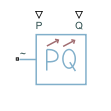• Library:
• Simscape / Electrical / Passive

•## Description

The Dynamic Load (Three-Phase) block models a balanced dynamic load for balanced three-phase supplies.

This table shows the relationship between the voltage from the supply, V, the active power at the P port, Pin, and the consumed active power of the block, Pactual.

Applicable Range of V ValuesApplicable Range of Pin ValuesCorresponding Consumed Active Power
$V>{V}_{\mathrm{min}}$${P}_{in}>{P}_{\mathrm{min}}$${P}_{actual}={P}_{in}$
${P}_{in}<{P}_{\mathrm{min}}$${P}_{actual}={P}_{\mathrm{min}}$
$V<{V}_{\mathrm{min}}$${P}_{in}>{P}_{\mathrm{min}}$The block models a load with an impedance equal to: $\begin{array}{l}R={V}_{\mathrm{min}}^{2}\frac{{P}_{actual}}{{P}_{actual}{}^{2}+{Q}_{actual}{}^{2}}\\ X={V}_{\mathrm{min}}^{2}\frac{{Q}_{actual}}{{P}_{actual}{}^{2}+{Q}_{actual}{}^{2}}\\ Z=\sqrt{{R}^{2}+{X}^{2}}\end{array}$
${P}_{in}<{P}_{\mathrm{min}}$${P}_{actual}={P}_{\mathrm{min}}$

Where:

The consumed reactive power of the block is equal to the Q port as long as the voltage from the supply is equal to or greater than the value specified for the Minimum supply voltage (phase-to-phase RMS) parameter. Otherwise, the block models a load with an impedance equal to:

`$\begin{array}{l}R={V}_{\mathrm{min}}^{2}\frac{{P}_{actual}}{{P}_{actual}{}^{2}+{Q}_{actual}{}^{2}}\\ X={V}_{\mathrm{min}}^{2}\frac{{Q}_{actual}}{{P}_{actual}{}^{2}+{Q}_{actual}{}^{2}}\\ Z=\sqrt{{R}^{2}+{X}^{2}}\end{array}$`

### Faults

The Dynamic Load (Three-Phase) block allows you to model an electrical fault as an open circuit. The block can trigger fault events at a specific time.

You can also choose whether to issue an assertion when a fault occurs by using the Reporting when a fault occurs parameter. The assertion can take the form of a warning or an error. By default, the block does not issue an assertion.

If the block is in a network that is compatible with the frequency-time simulation mode, you can perform a load-flow analysis on the network. A load-flow analysis provides steady-state values that you can use to initialize a machine.

For more information, see Perform a Load-Flow Analysis Using Simscape Electrical and Frequency and Time Simulation Mode.

## Limitations

If you connect this block to an inductive node or a current source, you must provide a large parasitic.

## Ports

### Input

expand all

Physical signal port associated with real power, specified as a scalar.

Physical signal port associated with reactive power, specified as a scalar.

### Conserving

expand all

Expandable three-phase electrical conserving port associated with the three-phase voltage.

## Parameters

expand all

Whether to model composite or expanded three-phase ports.

Composite three-phase ports represent three individual electrical conserving ports with a single block port. You can use composite three-phase ports to build models that correspond to single-line diagrams of three-phase electrical systems.

Expanded three-phase ports represent the individual phases of a three-phase system using three separate electrical conserving ports.

### Main

Minimum voltage, in phase-to-phase RMS, that the supply needs to give the block so that it acts as a dynamic load.

Minimum active power.

### Faults

Select `On` to enable fault modeling and expose the associated parameters in the Faults section, which enables you to select the reporting method and specify the time at which the fault triggers.

Choose whether to issue an assertion when a fault occurs:

• `None` — The block does not issue an assertion.

• `Warn` — The block issues a warning.

• `Error` — Simulation stops with an error.

#### Dependencies

To enable this parameter, set Enable open circuit fault to `On`.

Simulation time when the block enters the faulted state.

#### Dependencies

To enable this parameter, set Enable open circuit fault to `On`.

Conductance during the open-circuit state.

#### Dependencies

To enable this parameter, set Enable open circuit fault to `On`.

## Version History

Introduced in R2019a

expand all